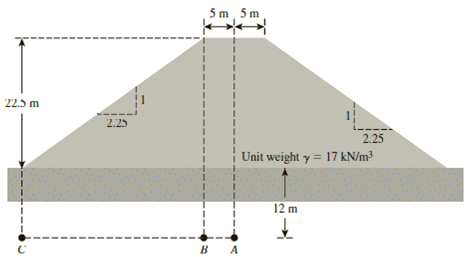Chapter 10, Problem 10.15PPrinciples of Geotechnical Enginee...

9th Edition
Braja M. Das + 1 other
ISBN: 9781305970939

Solutions

Chapter
SectionPrinciples of Geotechnical Enginee...

9th Edition
Braja M. Das + 1 other
ISBN: 9781305970939
Textbook Problem

For the embankment shown in Figure 10.45, determine the vertical stress increases at points A, B, and C.Figure 10.45

To determine

Calculate the increase in vertical stress at points A, B, and C.

Explanation

Given information:

The unit weight of the embankment soil (γ) is 17kN/m3.

The height of the embankment (H) is 22.5m.

The depth of the stress from ground surface (z) is 12m.

The top width of the embankment (B) is 10m.

The relation of horizontal to vertical is 2.25:1.

Calculation:

Consider the width of rectangular loading of the embankment as B1 and the width of triangular loading of the embankment as B2 in the cross sections.

Calculate the embankment loading (qo) as shown below.

qo=γH

Substitute 17kN/m3 for γ and 22.5m for H.

qo=17×22.5=382.5kN/m2

Calculate the bottom width of the triangular loading of the embankment (B2) as shown below.

B2=2.25H

Substitute 22.5m for H.

B2=2.25×22.5=50.6m

Calculate the increase in vertical stress (Δσz) using the relation as follows.

Δσz=qoI2

Here, I2 is the influence factor and as a function of B1z and B2z.

Stress increase at A:

Sketch the cross section of the loading diagram along point A as shown in Figure 1.

Refer to Figure 1.

The left and right side of the cross sections are equal.

Consider the left side of the cross section.

The width of rectangular loading of the embankment (B1) is 5m.

The width of triangular loading of the embankment (B2) is 50.6m.

Calculate the ratio B1z as shown below.

Substitute 5m for B1 and 12m for z.

B1z=512=0.417

Calculate the ratio B2z as shown below.

Substitute 50.6m for B2 and 12m for z.

B2z=50.612=4.217

Refer Figure 10.19 “Osterberg’s chart for determination of vertical stress due to embankment loading” in the Text Book.

Take the value of I2(L) as 0.47, for the values B1z of 0.417 and B2z of 4.217.

Hence, the value of I2(R) for right side of the cross section is 0.47.

Calculate the increase in vertical stress (Δσz) at point A due to the embankment load as shown below.

Δσz=q0(I2(L)+I2(R))

Substitute 382.5kN/m2 for qo, 0.47 for I2(L), and 0.47 for I2(R).

Δσz=382.5×(0.47+0.47)=359.55kN/m2

Hence, the increase in vertical stress at point A is 359.55kN/m2_.

Stress increase at B:

Sketch the cross section of the loading diagram along point B as shown in Figure 2.

Refer to Figure 2.

Consider the left side of the cross section.

The width of rectangular loading of the embankment (B1) is 0.

The width of triangular loading of the embankment (B2) is 50.6m.

Calculate the ratio B1z as shown below.

Substitute 0 for B1 and 12m for z.

B1z=012=0

Calculate the ratio B2z as shown below.

Substitute 50.6m for B2 and 12m for z.

B2z=50.612=4.217

Refer Figure 10.19 “Osterberg’s chart for determination of vertical stress due to embankment loading” in the Text Book.

Take the value of I2(L) as 0.425, for the values B1z of 0 and B2z of 4.217.

Consider the right side of the cross section.

The width of rectangular loading of the embankment (B1) is 10m.

The width of triangular loading of the embankment (B2) is 50

Still sussing out bartleby?

Check out a sample textbook solution.

See a sample solution

The Solution to Your Study Problems

Bartleby provides explanations to thousands of textbook problems written by our experts, many with advanced degrees!

Get Started

VocabularyState the meaning of the following terms: A component _____ A system _____

Engineering Fundamentals: An Introduction to Engineering (MindTap Course List)

What does the term 3 Vs refer to?

Database Systems: Design, Implementation, & Management

What is a business rule, and what is its purpose in data modeling?

Database Systems: Design, Implementation, & Management

What is comparison measurement?

Precision Machining Technology (MindTap Course List)

What e user applications? Suggest three examples that could boost user productivity.

Systems Analysis and Design (Shelly Cashman Series) (MindTap Course List)